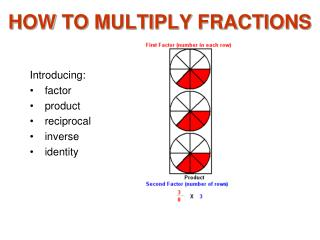DownloadDownload PresentationHOW TO MULTIPLY FRACTIONS

# HOW TO MULTIPLY FRACTIONS

Download Presentation## HOW TO MULTIPLY FRACTIONS

- - - - - - - - - - - - - - - - - - - - - - - - - - - E N D - - - - - - - - - - - - - - - - - - - - - - - - - - -
##### Presentation Transcript

1. HOW TO MULTIPLY FRACTIONS Introducing: • factor • product • reciprocal • inverse • identity

2. Multiply Fractions 1 The parts of this multiplication example are the first factor3/8 , and a second factor 3. There are 3 rows with 3/8 in each row.

3. Multiply Fractions 2 Multiplication is a form of addition. This picture shows that 3/8 is added 3 times. The product can be found by addition of like amounts: 3/8 + 3/8 + 3/8 = 9/8

4. Multiply Fractions 3 To calculate the product, write both factors in fraction form. Then multiply the numerators 3 and 3 for 9 in the product numerator and the denominators 8 and 1 for 8 in the product denominator.

5. Multiply Fractions 4 The product9/8 can be written in mixed form 1 1/8

6. Multiply Fractions 5 It is easy to tell the product 4 4/5 from this picture. Notice the 4 complete circles and the 2/5 + 2/5 circles for a product of 4 4/5.

7. Multiply Fractions 6 To calculate the product, write both factors in fraction form. Then multiply the numerators 12 and 2 for 24 in the product numerator and the denominators 5 and 1 for 5 in the product denominator.

8. Multiply Fractions 7 The same example, 2 2/5 x 2 using a rectangular array. The first factor 2 2/5, is shown by the red arrow - the horizontal distance. The second factor 2, is shown by the blue arrow - the vertical distance from the bottom. The product, 4 4/5 is enclosed by the yellow rectangle.

9. Multiply Fractions 8 This rectangular array shows the product of 4 1/2 and 1 1/2. Notice how each factor has been written in fraction form before multiplying. You can see in the picture that there are 27 fourths.

10. Multiply Fractions 9 Both factors are greater than 1. The product is greater than 4 x 1 but less than 5 x 2 by rounding down and rounding up both factors. So the product 6 3/4 makes sense.

11. Multiply Fractions 10 You can tell by the picture that there are 4 whole units, five 1/2 units, and one 1/4 units. The sum of the units is 4 + 5/2 + 1/4 = 6 3/4 .

12. Multiply Fractions 11 The second factor has been decreased to 1. The product has been decreased to 4 1/2 .

13. Multiply Fractions 12 When 1 is used as a factor, the product is equal to the other factor. One is called the identity for multiplication.

14. Multiply Fractions 13 The second factor has been decreased to 1/2. Notice the product has been decreased to 2 1/4. When one of the factors is smaller than 1, the product is smaller than the other factor.

15. Multiply Fractions 14 Both factors are less than 1. The product1/3 is smaller than either factor.

16. Multiply Fractions 15 The factors 1 1/4 and 4/5 are reciprocals. As you can see, multiplying 5/4 by 4/5 gives a product of 1. If you are asked to invert or write the reciprocal of 5/4 you will write 4/5.

17. Multiply Fractions 16 To find the reciprocal of a fraction, replace the denominator with the numerator and the numerator with the denominator. The reciprocal or inverse of 2/1 is 1/2.

18. Multiply Fractions 17 This picture shows 3 square units. Two 1/2 squares are selected. This gives a first factor of 2 and a second factor of 1/2. Added together, 1/2 and 1/2 squares give a sum of 1 unit.

19. Multiply Fractions 18 The factors 2 and 1/2 are reciprocals because their product is 1.